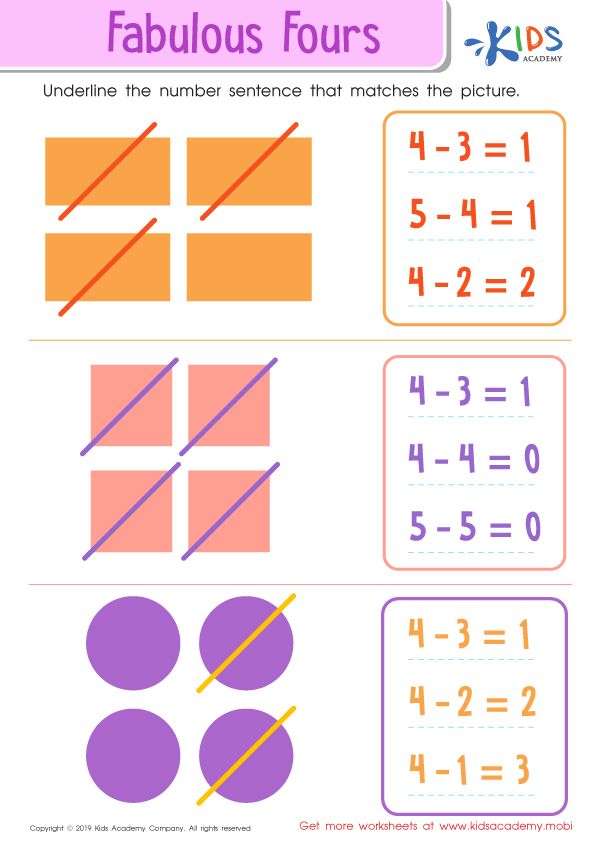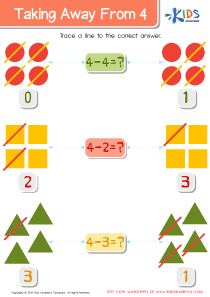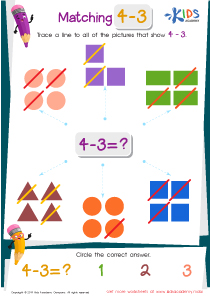# Math Lesson - Subtracting with 4's, Kindergarten

• ### Activity 1 / Fabulous Fours WorksheetBefore your children can go on to more complicated math theories and equations, they must first master the simpler ones like addition and subtraction of numbers. Use fun exercises and simple tests to see how well your kids understand math, and also help them get better at solving equations. In this worksheet, there are six different pictures. Help your kids count the objects in each picture, and then trace a line to all of the pictures that show the equation 3-2.

• ### Activity 2 / Taking Away from 4 Worksheet• ### Activity 3 / Matching 4 – 3 WorksheetBefore your children can go on to more complicated math theories and equations, they must first master the simpler ones like addition and subtraction of numbers. Use fun exercises and simple tests to see how well your kids understand math, and also help them get better at solving equations. In this worksheet, there are six different pictures. Help your kids count the objects in each picture, and then trace a line to all of the pictures that show the equation 4 – 3.

?